Foxtable(狐表)用户栏目专家坐堂 → 合并数据

共有564人关注过本帖树形打印复制链接

# 主题：合并数据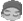Liangcai
1楼 | 信息 | 搜索 | 邮箱 | 主页 | UC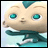加好友发短信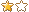Dim dlg As New OpenFileDialog
dlg.Filter = "Excel文件|*.xls;*.xlsx"
If dlg.ShowDialog =DialogResult.OK Then
Dim t As Table = Tables("学生缴费")
t.StopRedraw()
Dim Book As New XLS.Book(dlg.FileName)
Dim Sheet As XLS.Sheet = Book.Sheets(0)
Dim newcount As Integer = 0
Dim oldcount As Integer = 0
For n As Integer = 1 To Sheet.Rows.Count -1
Dim r As DataRow = t.DataTable.SQLFind("发票号 = '" & sheet(n, 0).text & "'")
If r Is Nothing Then
r = t.DataTable.AddNew()
newcount += 1
Else
oldcount += 1
End If
For i As Integer = 0 To sheet.Cols.Count -1
Dim cname As String = sheet(0, i).text
If t.Cols.Contains(cname) Then
r(cname) = sheet(n, i).Text
End If
Next
Next
msgbox("新增" & newcount & "    " & "更新旧数据" & oldcount)
t.ResumeRedraw()
t.Save()
End If
e.Form.controls("Table1").Table.Fill("Se lect * From {学生缴费}","数据库",True)

 下载信息  [文件大小：   下载次数： ]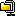点击浏览该文件:管理项目16.zip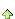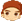2楼 | 信息 | 搜索 | 邮箱 | 主页 | UC加好友发短信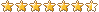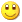Post By：2018/12/7 22:26:00 [只看该作者]

 ……    For n As Integer = 1 To Sheet.Rows.Count -1        Dim r As DataRow = t.DataTable.SQLFind("发票号 = '" & sheet(n, 3).text & "'")        If r Is Nothing Then            r = t.DataTable.AddNew()            newcount += 1        Else            oldcount += 1        End If        For i As Integer = 0 To sheet.Cols.Count -1            Dim cname As String = sheet(0, i).text            If t.Cols.Contains(cname) Then                r(cname) = sheet(n, i).Text            End If        Next        r.save    Next……Liangcai
3楼 | 信息 | 搜索 | 邮箱 | 主页 | UC加好友发短信Post By：2018/12/7 23:21:00 [只看该作者]

 谢谢Liangcai
4楼 | 信息 | 搜索 | 邮箱 | 主页 | UC加好友发短信Post By：2018/12/12 19:09:00 [只看该作者]

 老师文件比较大，怎么加进度条？请老师指教，谢谢！5楼 | 信息 | 搜索 | 邮箱 | 主页 | UC加好友发短信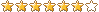Post By：2018/12/12 20:38:00 [只看该作者]

 以下是引用Liangcai在2018/12/12 19:09:00的发言：老师文件比较大，怎么加进度条？请老师指教，谢谢！